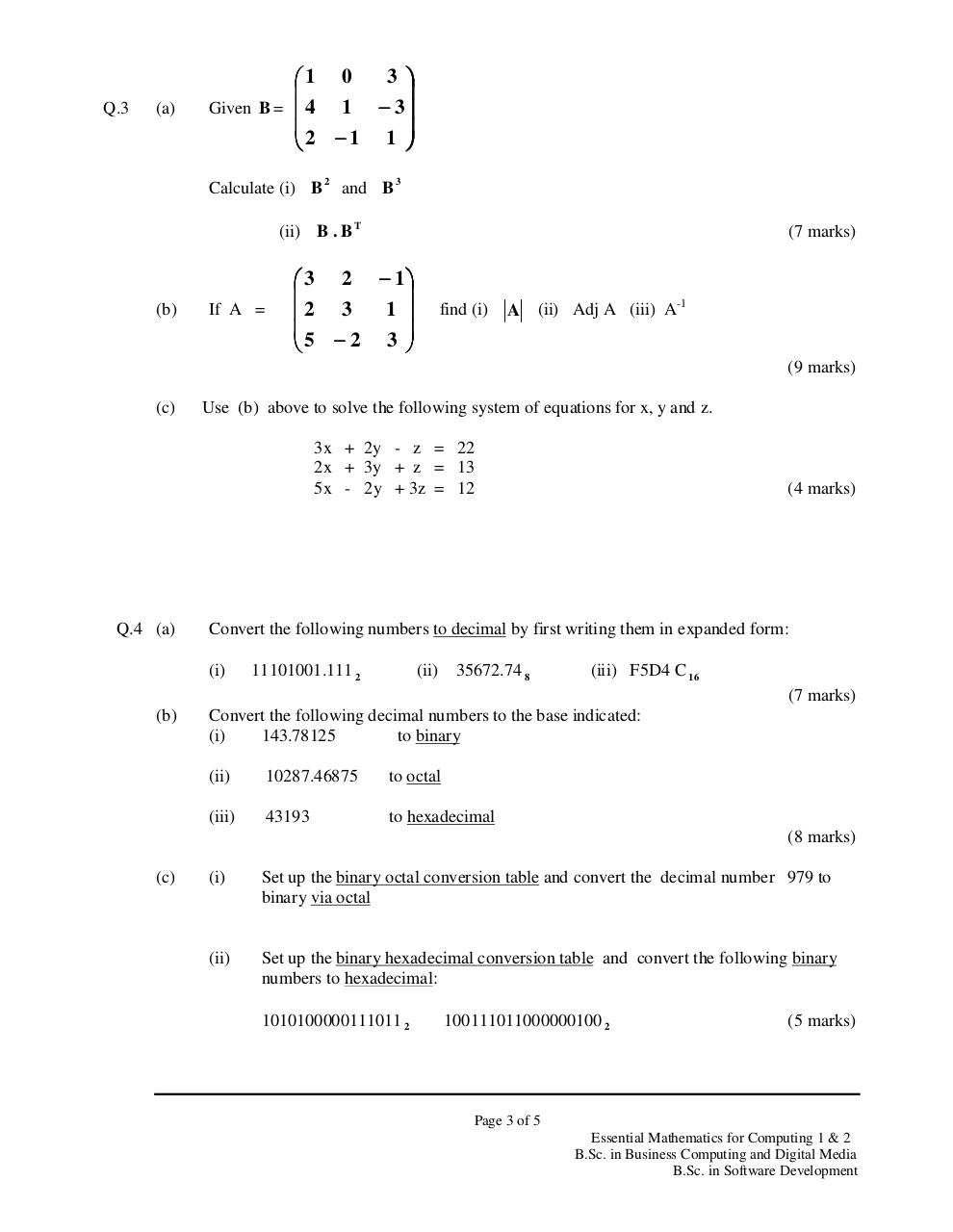# Maths (Summer 2010).PDFPage 1 2 3 4 5 6

#### Text preview

Q.3

(a)

3 
1 0

Given B =  4
1 − 3
2 − 1 1 

Calculate (i) B 2 and B 3
(ii) B . B T

(b)

If A =

(7 marks)

 3 2 − 1

1 
2 3
5 − 2 3 

find (i)

A

(9 marks)

(c)

Use (b) above to solve the following system of equations for x, y and z.
3x + 2y - z = 22
2x + 3y + z = 13
5x - 2y + 3z = 12

Q.4 (a)

(4 marks)

Convert the following numbers to decimal by first writing them in expanded form:
(i)

11101001.111 2

(ii)

35672.74 8

(iii) F5D4 C 16
(7 marks)

(b)

Convert the following decimal numbers to the base indicated:
(i)
143.78125
to binary
(ii)

10287.46875

to octal

(iii)

43193

(8 marks)

(c)

(i)

Set up the binary octal conversion table and convert the decimal number 979 to
binary via octal

(ii)

Set up the binary hexadecimal conversion table and convert the following binary